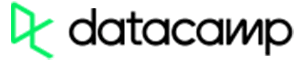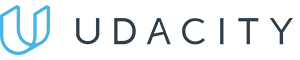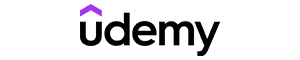Code has been added to clipboard!

# Operator

Published Aug 9, 2017
Updated Oct 2, 2019

## JavaScript Operator: Main Tips

• In JavaScript, operators are used for assigning values, performing arithmetic operations, and executing other useful tasks.
• Certain operators should be used sparingly since they can slow down or complicate the execution of your script.

## Operator Types

Some JavaScript operators get used more than others. That's only natural: we encounter some needs more often in our daily work.

Actions like calculation, comparison, and some others are particularly common. This is why JavaScript provides a variety of operators to suit our needs. We will now present you with the six most important types of operators, and provide examples for better understanding.

### Arithmetic

You should remember arithmetic operations from your school years: they're the same omnipresent calculations that you start learning in the first grade. In JavaScript, you have the operators and operands.

Simply put, operators refer to the signs of addition (`+`), subtraction (`-`), division (`/`) and multiplication (`*`). Operands refer to the numbers that are used in the aritmetic calculations.

Let's imagine that the variable b has a value of 8 assigned to it:

Operator Description Example Result in b Result in a
+ Add a = b + 2 b = 8 a = 10
- Subtract a = b - 2 b = 8 a = 6
* Multiply a = b * 2 b = 8 a = 16
/ Divide a = b / 2 b = 8 a = 4
% Modulus (remainder of division) a = b % 2 b = 8 a = 0
++ Increment a = ++b b = 9 a = 9
a = b++ b = 9 a = 9
-- Decrement a = --b b = 7 a = 7
a = b-- b = 7 a = 7

### Assignment

Using assignment operators, you give variables a value. In other words, the assigment operator is an equal sign (`=`). It is used to set the value of the right operand to the left operand.

Let's imagine we have to declare two values like this: `a = 10` and `b = 8`. The table below will use these two variables with values to explain assignment operators:

Operator Example Same As Result in a
= a = b a = b a = 8
+= a += b a = a + b a = 18
-= a -= b a = a - b a = 2
*= a *= b a = a * b a = 80
/= a /= b a = a / b a = 1
%= a %= b a = a % b a = 2Pros
• Easy to use with a learn-by-doing approach
• Offers quality content
• Gamified in-browser coding experience
• The price matches the quality
• Suitable for learners ranging from beginner to advanced
Main Features
• Free certificates of completion
• Focused on data science skills
• Flexible learning timetablePros
• Simplistic design (no unnecessary information)
• High-quality courses (even the free ones)
• Variety of features
Main Features
• Nanodegree programs
• Suitable for enterprises
• Paid Certificates of completionPros
• Easy to navigate
• No technical issues
• Seems to care about its users
Main Features
• Huge variety of courses
• 30-day refund policy
• Free certificates of completion

### String

The `+` and the JavaScript `+=` operators can also be used for concatenating (adding) strings.

To put it simply, these concatenation operators `+=` and `+` are applied when developers wish to add the values of multiple strings. As a result, a new string is produced that represents the calculated value.

Let's imagine that we have declared three values like this: `string1 = "Hey, "`, `string2 = "Joe"`, `string3 = ""`.

The table below will use these three variables with values to explain string operators:

Operator Example string1 string2 string3
+ string3 = string1 + string2 "Hey, " "Joe" "Hey, Joe"
+= string1 += string2 "Hey, Joe " "Joe" ""

### Comparison

Comparison operators allow you to get a boolean value of true or false by comparing two variables for equal value or both value and type.

Once the JavaScript `==` is applied, it forcefully transforms two compared operands to the same type. On the other hand, JavaScript `===` performs the same function a little differently: it checks whether the two operands are of the same type. If they are, JavaScript `===` returns true.

Let's imagine that we have declared a value like this:` a = 8`. The table below will use this variable to explain comparison operators:

Operator Description Comparing Returns
== equal value a == 8 false
a == 8 true
=== equal value and equal data type a === "8" false
a === 8 true
!= not equal a != 8 true
!== not equal value or not equal data type a !== "8" true
a !== 8 false
> greater than a > 8 false
< less than a < 8 true
>= greater than or equal to a >= 8 false
<= less than or equal to a <= 8 true

### Logical

Using logical operators, you can determine the logic between operators and variables. Therefore, the logical operators are applied to get a boolean value (either true or false).

Let's imagine that we have declared a value like this:` a = 6 and b = 3`. The table below will use these two variables with values to explain logical operators:

Operator Description Example
&& and (a < 10 && b > 2) is true
|| or (a === 8 || b === 8) is false
! not !(a === b) is true

### Bitwise

JavaScript bitwise operators are meant to work with 32-bit numbers. For instance, the number 8 has a binary representation of 1000. Numeric operands are turned to 32-bit values when used with these operators. After the JavaScript bitwise operators are done, the result is converted back into a regular JavaScript number.

Operator Description Example Same as Result Decimal
^ XOR a = 8 ^ 1 0101 ^ 0001 0100 4
| OR a = 8 | 1 0101 | 0001 0101 8
& AND a = 8 & 1 0101 & 0001 0001 1
~ NOT a = ~ 8 ~0101 1010 10
>> Right shift a = 8 >> 1 0101 >> 1 0010 2
<< Left shift a = 8 << 1 0101 << 1 1010 10

Note: Above example uses 4 bits unsigned example. However, JavaScript would use 32-bit signed numbers. Therefore, ~8 would not return 10 in JavaScript. The result would be -6.

## Other Operators

We have covered the biggest types of operators that get used fairly often. Now, there are also operators that often prove useful, but don't belong to any bigger groups. We will discuss six of them, providing code examples. Understanding how these JavaScript operators work will make you more flexible in your work.

#### Conditional (Ternary)

Ternary operator assigns a value to a variable if a condition is met. See a snippet below to understand the syntax rules:

`exampleVar = (condition) ? val1:val2;`

To get a better understanding, let's try an example:

`canVote = (age < 18) ? "Not old enough":"Old enough";`

A check on the variable called `age` is performed, and if its value is less than 18, the value of the variable `voteable` is going to be `Not old enough`, otherwise its value will be `Old enough`.

#### typeof

The `typeof` operator is used to return the type of variables, objects, functions or expressions:

Example
``````typeof "Joe"                    // Will return string
typeof 2                        // Will return number
typeof NaN                      // Will return number
typeof true                     // Will return boolean
typeof [9, 8, 7, 6]             // Will return object
typeof {name:'Joe', age:32}     // Will return object
typeof new Date()               // Will return object
typeof function () {}           // Will return function
typeof exampleVar               // Will return undefined (if exampleVar has not been declared)
typeof null                     // Will return object``````

Notice how:

• Data type of `NaN` is number.
• Data type of an `array` is object.
• Data type of a `Date` is object.
• Data type of `null` is object.
• Data type of an undefined variable is `undefined`.

Note: typeof cannot be used for defining if a JavaScript object is a date or an array.

#### delete

The operator `delete` is used to delete objects from operators:

Example
``````var user = {
name: "Joe",
age: 32
};
delete user.age;``````

Using this operator you delete both the property and its value. After that, the property cannot be accessed in any way, unless a similar one is added back.

This operator does not affect variables or functions. It's only designed to work on object properties.

Note: Do NOT use this operator on pre-defined JavaScript properties. It may crash your application.

#### in

The `in` operator is used to check whether a property is in the specified object, returning the value either true or false:

Example
``````var car = ["BMW", "Audi", "Volvo"];
"Audi" in car
1 in car
2 in car
5 in car
"length" in car
// Objects
var user = {
name: "Joe",
age: 32
};
"name" in user
"age" in user
// Predefined objects
"length" in String
"NaN" in Number
"PI" in Math``````

#### instanceof

The `instanceof` operator is used to check if a specified object is an instance of a specified object, returning the value of either true or false. Therefore, the JavaScript instanceof will return a boolean value.

Example
``````var car = ["BMW", "Audi", "Volvo"];
car instanceof Object;
car instanceof Array;
car instanceof Number;
car instanceof String;``````

#### void

The `void` operator is used to evaluate an expression and return undefined. The JavaScript void is often used for obtaining the undefined primitive value, by using `void(0)` (useful for evaluating an expression without using the return value).

Example
``````<a href="javascript:void(0);"> Link with no destination</a>
<a href="javascript:void(document.body.style.backgroundColor='green');">Click to make the background red</a>``````

Days
Hours
Minutes
Seconds